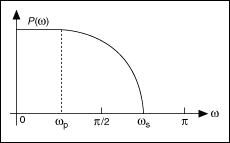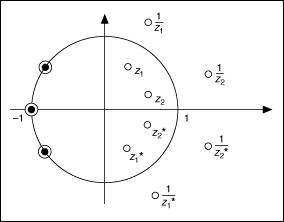# Designing the Product P0(z) (Advanced Signal Processing Toolkit)

LabVIEW 2014 Advanced Signal Processing Toolkit Help

Edition Date: June 2014

Part Number: 372656C-01

»View Product InfoDownload Help (Windows Only)

The auxiliary function P0(z) denotes the product of G0(z) and H0(z), as shown in the following equation:

P0(z) = G0(z) + H0(z)

You usually use one of the following three types of filters for P0(z).

• Maximally-flat
• General equiripple halfband
• Positive equiripple halfband

The maximally-flat filter is defined by the following equation:

P0(z) = (1 + z–1)2pQ(z)

In the Wavelet Design Express VI, the Zero pairs at(P0) control on the Configure Wavelet Design dialog box specifies the value of the parameter p, which determines the number of zeros placed at π on the unit circle. The more the zeros at, the smoother the corresponding wavelet. The value of p also affects the transition band of the frequency response. A large value of p results in a narrow transition band. In the time domain, a narrower transition band implies more oscillations in the corresponding wavelet. When you specify a value for the parameter p, you can review the frequency response shown on the right-hand side of the Configure Wavelet Design dialog box.

In a general equiripple halfband filter, halfband refers to a filter in which ωs + ωp = π, where ωs denotes the stopband frequency and ωp denotes the passband frequency, as shown in the following figure.The positive equiripple halfband filter is a special case of general equiripple halfband filters. The Fourier transform of this type of filter is always nonnegative. Positive equiripple halfband filter is appropriate for orthogonal wavelets because the auxiliary function P0(z) must be nonnegative.

Use the P0type control to specify the P0(z) type. When Wavelet Type is set to Orthogonal, you can set P0(z) either to Maxflat (default), for a maximally-flat filter, or to Positive Equiripple. When Wavelet Type is set to Biorthogonal, you can set P0(z) to Maxflat (default), Positive Equiripple, or General Equiripple.

Because all filters, including P0(z), G0(z), and H0(z), are real-valued finite impulse response (FIR) filters, the zeros of these filters are mirror-symmetric about the x-axis in the z-plane. Therefore, for any zero zi, a corresponding complex conjugate zi* always exists. If zi is complex, meaning that if zi is located off of the x-axis, you always can find a corresponding zero on the other side of the x-axis, as shown in the following figure. As a result, you only need to see the top half of the z-plane to see all of the zeros that are present. After you select zi, the Wavelet Design Express VI automatically includes the complex conjugate zi*.Two parameters are associated with equiripple filters—# of taps and Passband. Use the # of taps control to define the number of coefficients of P0(z). Because P0(z) is a type-I FIR filter, the length of P0(z) must be odd. Use the Passband control to define the normalized passband frequency, ωp, of P0(z). The value of ωp must be less than 0.5. Longer filters improve the sharpness of the transition band and the magnitude of the attenuation in the stopband at the expense of extra computation time for implementation.